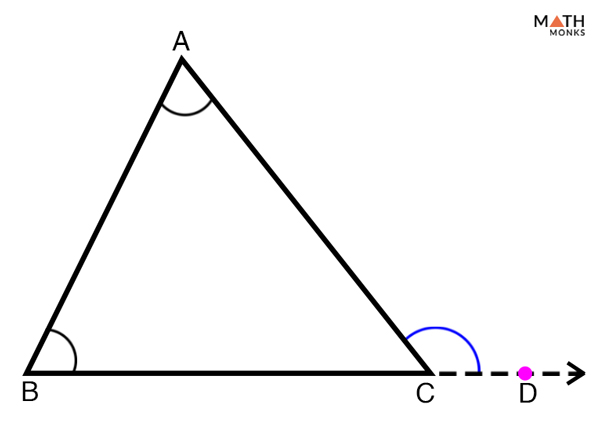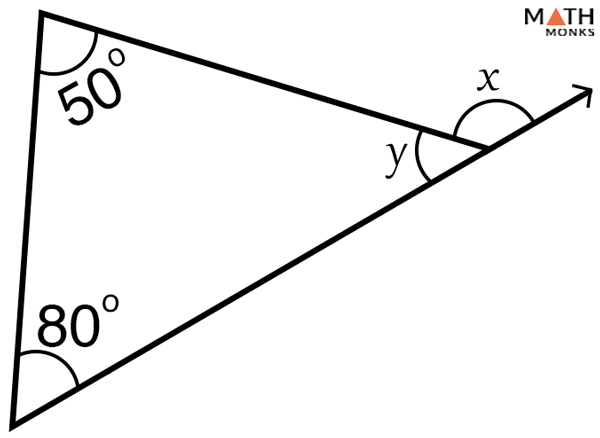# Exterior Angle of a Triangle

## What is the Exterior Angle of a Triangle

The exterior angle of a triangle is the angle formed between one side and the extension of its adjacent side.

Shown below is the ΔABC where ∠ACD is the exterior angle formed by the side AC and the extension of the side BC to D.

## How Many Exterior Angles Does a Triangle Have

Every triangle has three exterior angles, two at each vertex.

## Properties

1. An exterior angle of a triangle is equal to the sum of the two opposite interior angles, thus an exterior angle is greater than any of its two opposite interior angles; for example, in ΔABC, ∠5 = ∠a + ∠b
2. The sum of an exterior angle and its adjacent interior angle is equal to 180 degrees; for example, ∠5 + ∠c = 180°
3. All exterior angles of a triangle add up to 360° (taken one angle at each vertex); ∠1 + ∠3 +∠5 = 360°
4. The two exterior angles at each vertex are equal (vertically opposite angles); ∠1 = ∠2, ∠3 =∠4, & ∠5 = ∠6

## Triangle Exterior Angle Theorem

### Triangle Exterior Angle Theorem ProofProve Triangle Exterior Angle Theorem

To prove:

∠ACD = ∠ABC + ∠CAB

Proof:

Given: ΔABC, ABD is a line segment
∠ACD + ∠BCA = 180° … (1) [∠ACD & ∠BCA form a linear pair, they are supplementary]
∠ABC + ∠BCA + ∠CAB = 180° …. (2) [Angle Sum Property of a Triangle]
Substituting (1) in (2), we get,
∠ACD + ∠BCA = ∠ABC + ∠BCA + ∠CAB
∠ACD = ∠ABC + ∠CAB
Hence Proved

Given below are some solved examples to understand the concept.

## How to Find the Exterior Angles of a Triangle

Here, we will apply the exterior angle theorem to find the missing interior or exterior angles in a triangle.In the given triangle, if m∠1 = 40° and m∠2 = 80°, find m∠4.

Solution:

As we know, from the exterior angle theorem,
m∠4 = m∠1 + m∠2, here m∠1 = 40°, m∠2 = 80°
m∠4 = 40° + 80° = 120°Solve for x.

Solution:

As we know, from the exterior angle theorem,
(x – 2) + (2x + 10) = 140°
=> 3x + 8 = 140°
=> 3x = 132
=> x = 44

If the measure of the exterior angle is (5x – 10) degrees, and the measure of the two remote interior angles are 30 degrees and (x + 20) degrees, find x.

Solution:

As we know, from the exterior angle theorem,
(5x – 10) = 30° + (x + 20)
5x – x = 30° + 20° + 10°
4x = 60°
x = 15°Calculate values of x and y in the given triangle.

Solution:

In the given triangle, x is the exterior angle and y is the interior angle
As we know, from the exterior angle theorem,
x = 80° + 50° = 130°
Now, as x and y are linear pairs that add up to 180°
Thus,
x + y = 180°, here x = 130°
130° + y = 180°
y = 180° – 130° = 50°